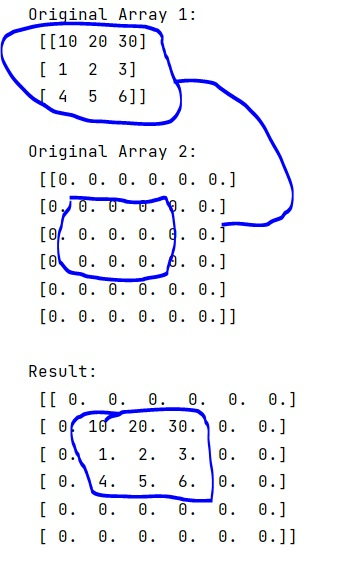# How to copy NumPy array into part of another array?

Learn, how to copy NumPy array into part of another array in Python?
Submitted by Pranit Sharma, on February 19, 2023

NumPy is an abbreviated form of Numerical Python. It is used for different types of scientific operations in python. Numpy is a vast library in python which is used for almost every kind of scientific or mathematical operation. It is itself an array which is a collection of various methods and functions for processing the arrays.

## Copying NumPy array into part of another array

Suppose that we are given a numpy array with a fixed size and we are creating a larger array. We need to efficiently copy the smaller array into some part of the larger array. Suppose that we are given a 3 X 3 array and the size of the larger array is 6 X 6 and we need to fix the smaller array into some specific position in the larger array and the rest of the positions will be filled with zeros.

For this purpose, we will create two arrays first, the smaller one with the actual elements and the larger array with all the elements as 0. We will then specify a position by indexing the larger array and assigning that position to the smaller array's values.

Let us understand with the help of an example,

## Python code to copy NumPy array into part of another array

```# Import numpy
import numpy as np

# Creating two numpy arrays
arr1 = np.array([[10, 20, 30],[1,2,3],[4,5,6]])
arr2 = np.zeros((6,6))

# Display original arrays
print("Original Array 1:\n",arr1,"\n")
print("Original Array 2:\n",arr2,"\n")

# Fixing smaller array into larger array
# at some specific position
arr2[1:4, 1:4] = arr1

# Display result
print("Result:\n",arr2)
```

Output: